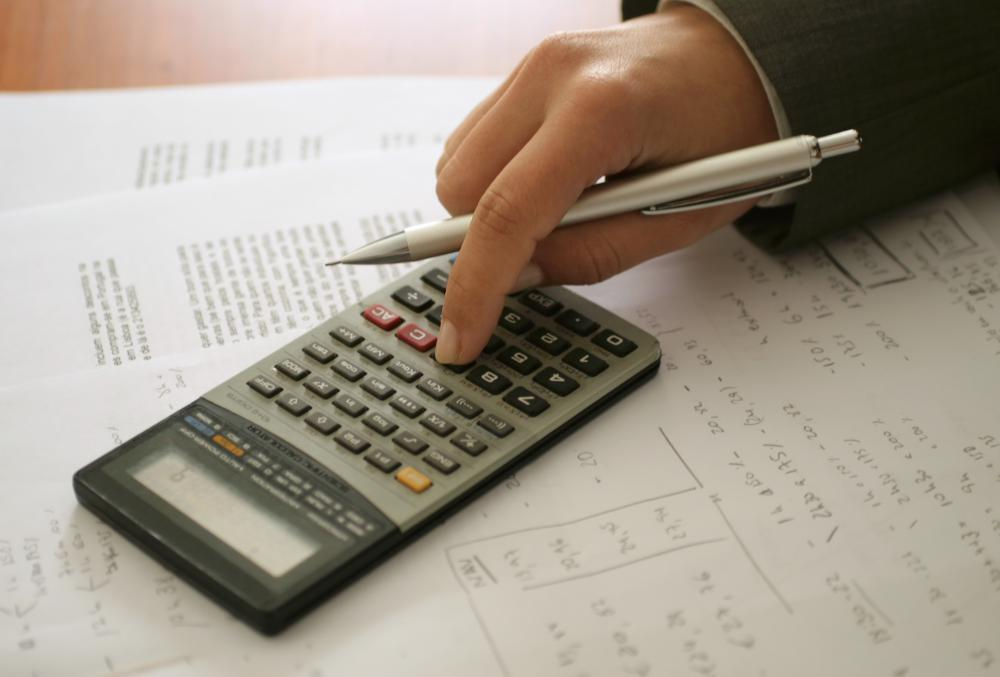# How is Deposit Interest Calculated?

A. Rohlandt
A. RohlandtWhere the calculation of deposit interest can get confusing is in what is known as compounding.

Deposit interest, or the money paid as an incentive for saving or investing is calculated using a straightforward formula where the balance is multiplied by the percentage of the interest expressed as a decimal. For example, 10 percent interest on \$1,000 US Dollars (USD) would be calculated as \$1,000 USD x .10 or \$100 USD. Where the calculation of deposit interest can get confusing is in what is known as compounding. Compounding is the process of taking the interest that has been earned during a certain time period and adding it to the balance, then calculating again for the next period starting with the increased balance.

How the balance is compounded is stated in the terms of the certificate of deposit (CD) or the rules of the savings account. Also requiring definition is how the balance will be reckoned; for daily compounding, this is generally understood to be the balance of the account at the end of the previous day. For other terms of compounding i.e., quarterly, monthly or other, this is important because it can affect the amount of interest earned on a deposit.

In the example used above, for daily compounding, the formula is changed to: balance x interest rate/365 + balance = new balance. Substituting, we find (\$1,000 USD x .10/365) + \$1,000 USD = \$1,000.27 USD. The new balance of \$1000.27 after one day is then placed at the beginning of the equation for the next day. Compounding daily would yield a balance of \$1105.16 USD after one year. If this were computed as simple annual interest, the balance would be \$1,100 USD.

For other compounding variants, the only difference would be by what number the interest rate would be divided; for monthly compounding it would be divided by 12, for quarterly compounding by four. So, for monthly compounding, the calculation would be thus: (\$1,000 USD x .10/12) + \$1,000 USD. At the end of 12 months, the balance would be \$1,104.71 USD. Quarterly compounding would yield a balance of \$1,103.81 USD. It can be seen that the more frequently compounding occurs, the higher the deposit interest earned.

Some institutions or account providers might stipulate conditions on how the balance is averaged to yield a lower amount on which the deposit interest is calculated. If a CD stipulated that the monthly compounding would occur on a balance that is equal to the average of the previous three months' balances, that would result in a lower amount multiplied by the interest rate and therefore, a lower return. A similar tactic is used by credit card companies to increase the balance on which interest charges are computed for their card holders.

## You might also Like